# Entanglement and Quantum Phase Transition in Matrix Product

• Slides: 20Entanglement and Quantum Phase Transition in Matrix Product Systems S. Alipour Department of Physics, Sharif University of Technology Tehran, Iran International Iran Conference on Quantum Information, 7 -10 Sept. 2007, Kish Island 0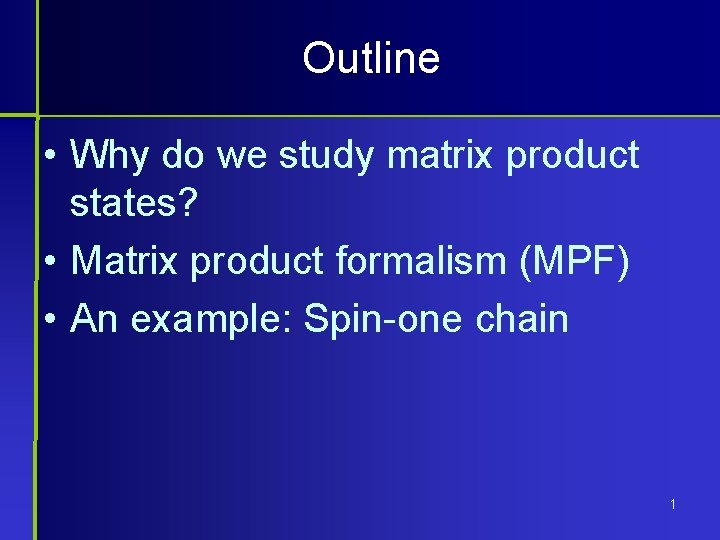Outline • Why do we study matrix product states? • Matrix product formalism (MPF) • An example: Spin-one chain 1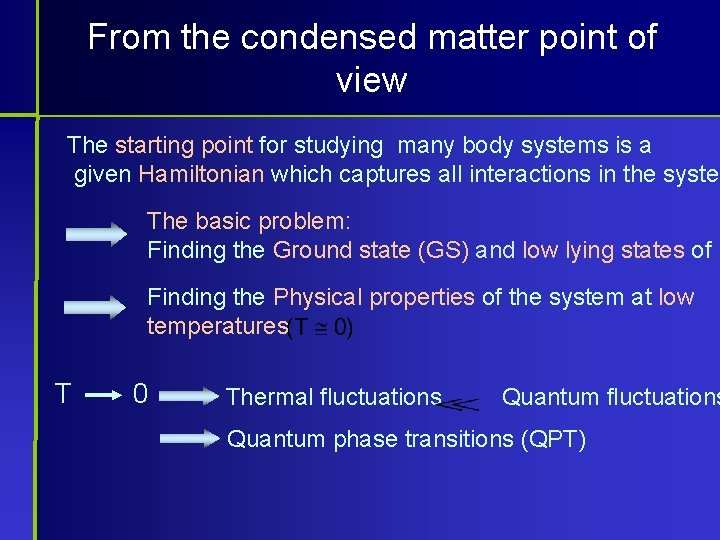From the condensed matter point of view The starting point for studying many body systems is a given Hamiltonian which captures all interactions in the system The basic problem: Finding the Ground state (GS) and low lying states of H Finding the Physical properties of the system at low temperatures T 0 Thermal fluctuations Quantum phase transitions (QPT)From the condensed matter point of view Physical properties of interest: • • Critical exponents Correlation length N-point functions … Unfortunately solving these problems is extremely difficult. There are only a few rare examples: • XX chain (free fermion) • Heisenberg chain (Bethe ansatz) 3From the quantum information point of view The emphasis is on the properties of given states. A very important property: Entanglement between particles The state which has enough amount of multi-partite entangleme can be used as an initial state in a quantum computer. The basic problem: To find a physical system (H) whose GS is this state. 4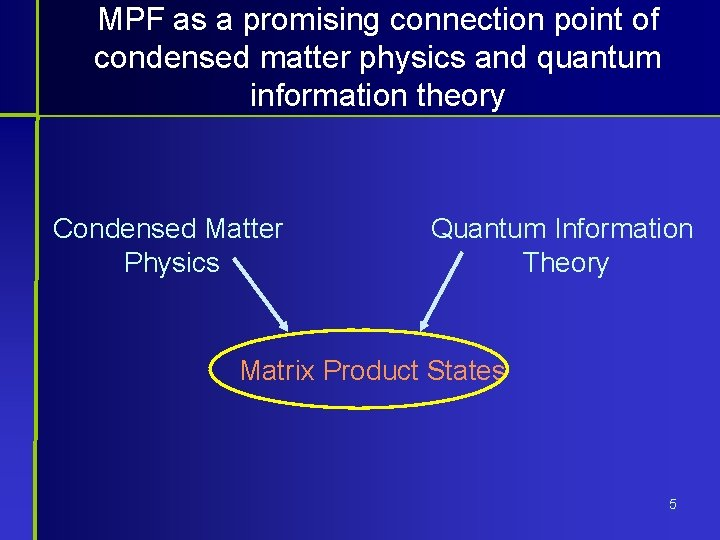MPF as a promising connection point of condensed matter physics and quantum information theory Condensed Matter Physics Quantum Information Theory Matrix Product States 5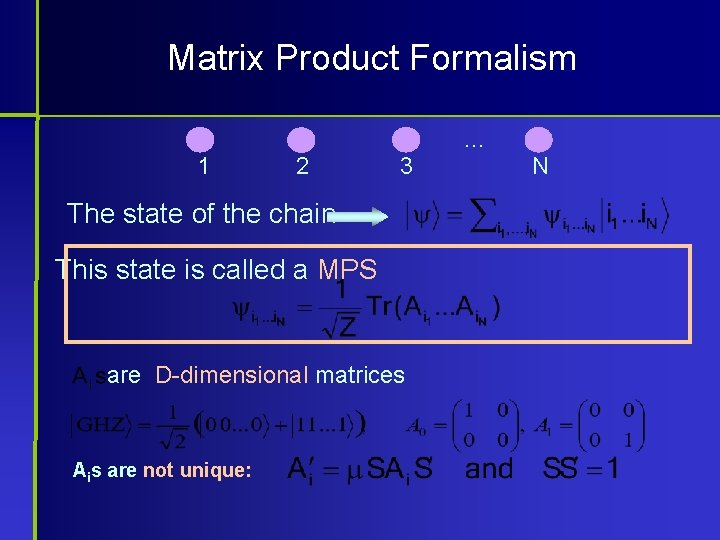Matrix Product Formalism … 1 2 3 The state of the chain This state is called a MPS are D-dimensional matrices Ais are not unique: N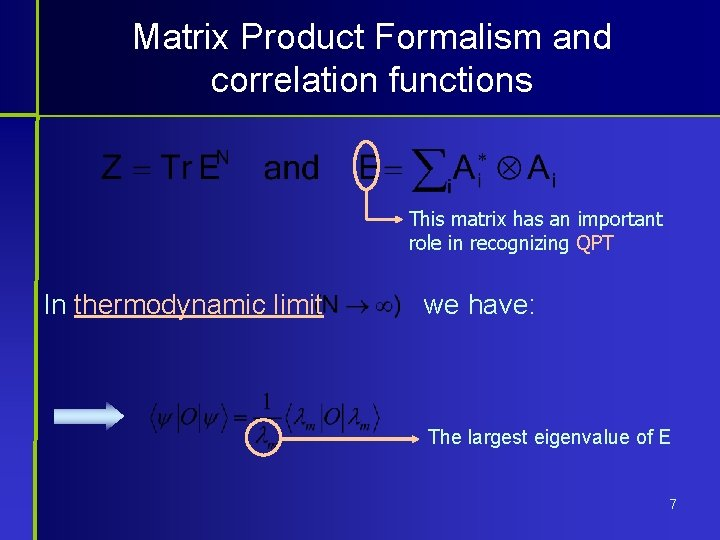Matrix Product Formalism and correlation functions This matrix has an important role in recognizing QPT In thermodynamic limit we have: The largest eigenvalue of E 7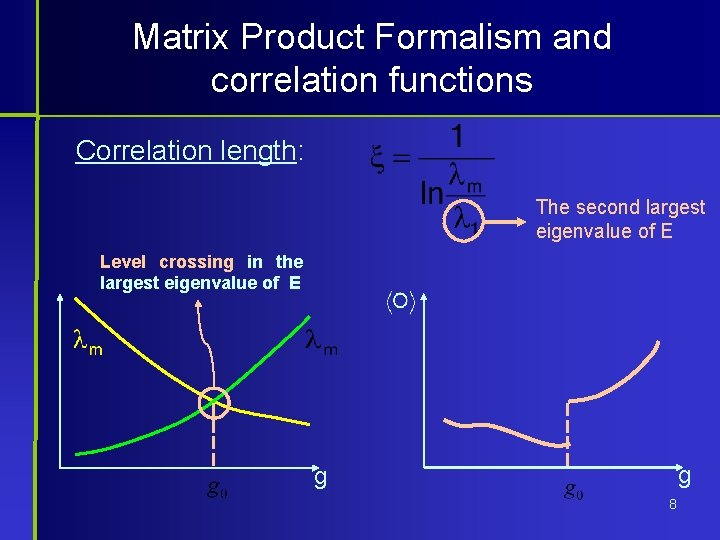Matrix Product Formalism and correlation functions Correlation length: The second largest eigenvalue of E Level crossing in the largest eigenvalue of E g g 8Systematic construction of MPS: Symmetries Tensor-product Symmetry: R is a d-dimensional representation of the symmetry operator U is a D-dimensional representation of the symmetry 9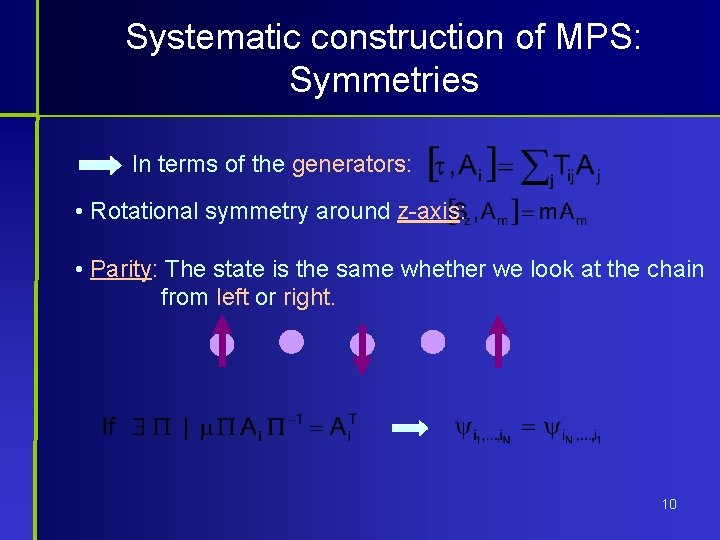Systematic construction of MPS: Symmetries In terms of the generators: • Rotational symmetry around z-axis: • Parity: The state is the same whether we look at the chain from left or right. 10The Hamiltonian • How we can construct the Hamiltonian? H is positive and is the GS of H 11Example: A spin-one chain I. Affleck et al. PRL (1987) Full rotational symmetry Rotation around z-axis onlyEntanglement of two spins at sites 1 and r as a function of their distance and the coupling g is a QPT point Entanglement exist up to a range of 30 0. 02 13Negativity of two spins with different distances a a function of system size 14Negativity of two spins as a function of their distance for a ring of size N=40 15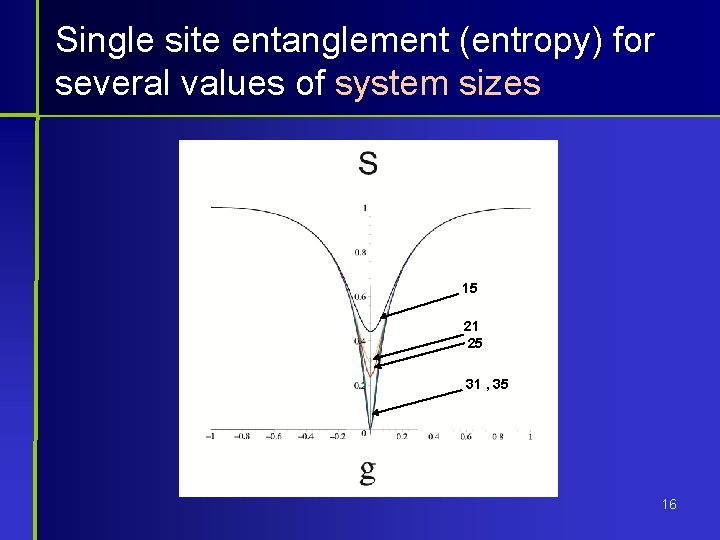Single site entanglement (entropy) for several values of system sizes 15 21 25 31 , 35 16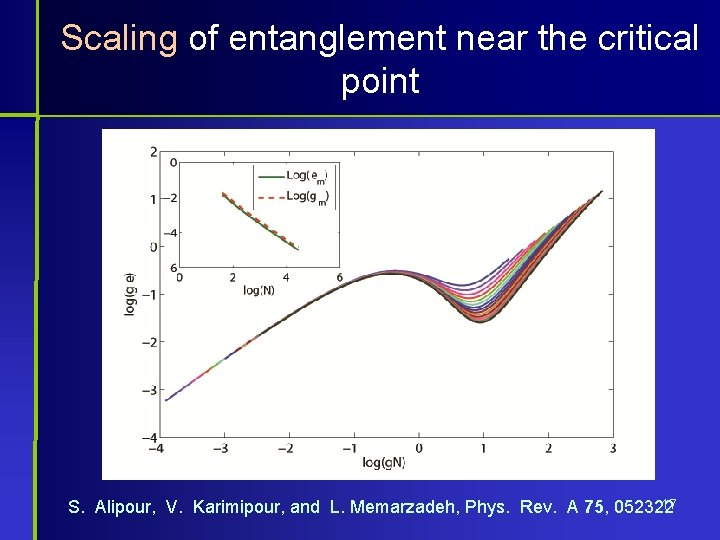Scaling of entanglement near the critical point 17 S. Alipour, V. Karimipour, and L. Memarzadeh, Phys. Rev. A 75, 052322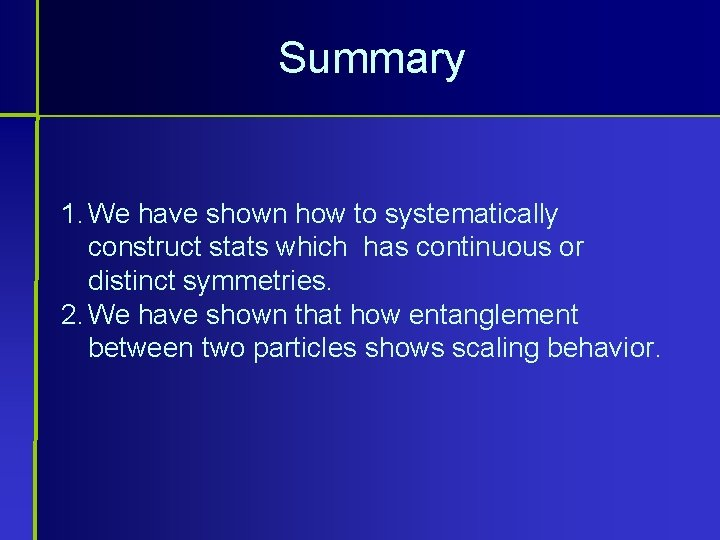Summary 1. We have shown how to systematically construct stats which has continuous or distinct symmetries. 2. We have shown that how entanglement between two particles shows scaling behavior.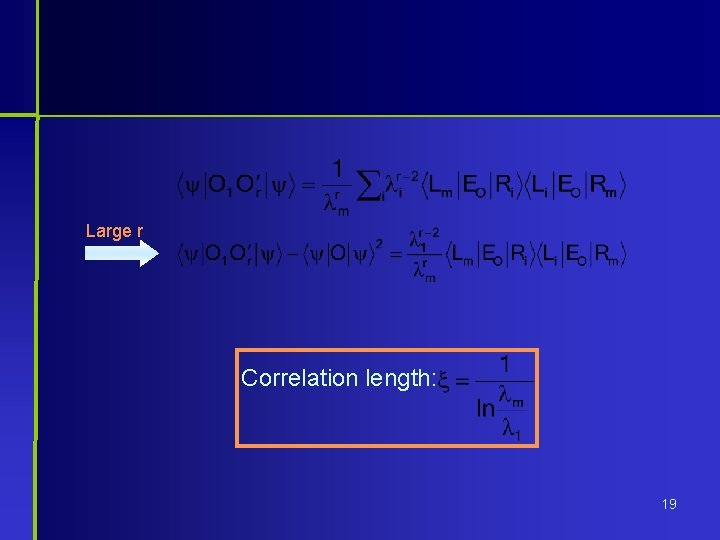Large r Correlation length: 19